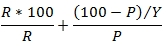# XLeratorDB/financial Documentation

Updated: 03 Mar 2017

Use the scalar function ADJCURRYIELD to calculate the adjusted current yield for a bond. The formula for the adjusted current yield is:R = the coupon rate expressed as decimal (1% = .01) P = the clean price Y = the year fraction from settlement date to maturity date.
Syntax
<@Settlement, datetime,>
,<@Maturity, datetime,>
,<@Price, float,>
,<@Rate, float,>
,<@Basis, nvarchar(4000),>)
Arguments
 Input Name Description @Settlement Settlement date of the transaction. @Settlement must be of type datetime or of a type that implicitly converts to datetime. @Maturity Settlement date of the transaction. @Settlement must be of type datetime or of a type that implicitly converts to datetime. @Price Clean price. @Price must of a type float or of a type that implicitly converts to float. @Rate Coupon rate of the instrument expressed as decimal (1% = .01). @Rate must be of type float or of a type that implicitly converts to float. @Basis The day-count convention used in the calculation of the year fraction from @Settlement to @Maturity. See YEARFRAC for valid day-count conventions.
Return Types
float
Remarks
• If @Settlement is NULL then @Settlement = GETDATE()
• If @Maturity is NULL then @Maturity = GETDATE()
• If @Price is NULL then @Price = 100
• If @Rate is NULL then @Rate = 0
• If @Basis is NULL then @Basis = 0
• Available in XLeratorDB / financial 2008 only
Examples
'2017-03-03'    --@Settlement
,'2024-04-01'    --@Maturity
,101.94          --@Price
,0.02575        --Rate
,'ACTUAL'        --@Basis

This produces the following result.Copyright 2008-2021 Westclintech LLC         Privacy Policy        Terms of Service